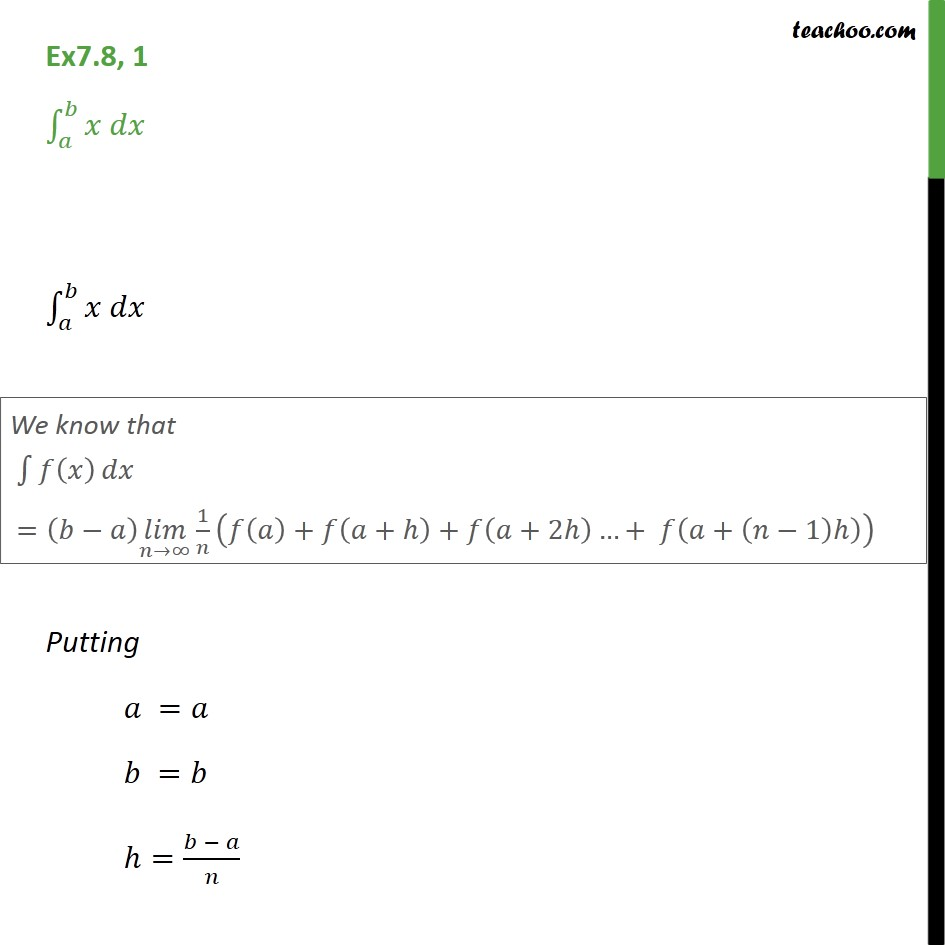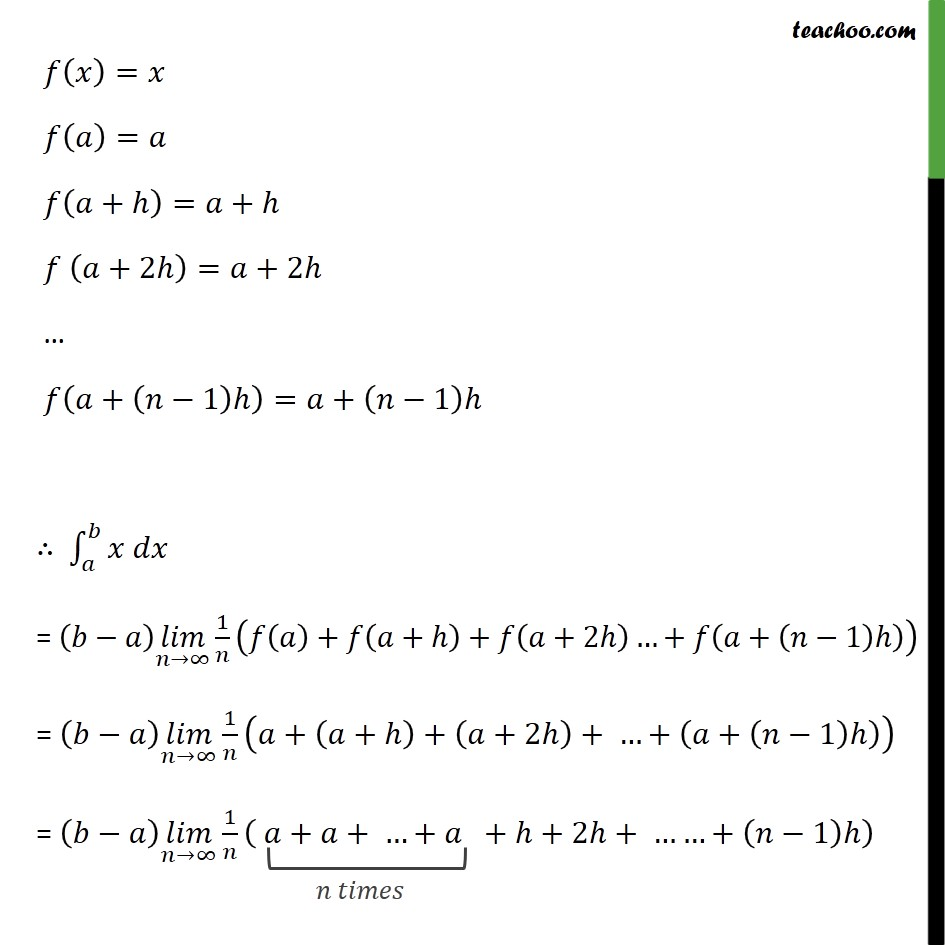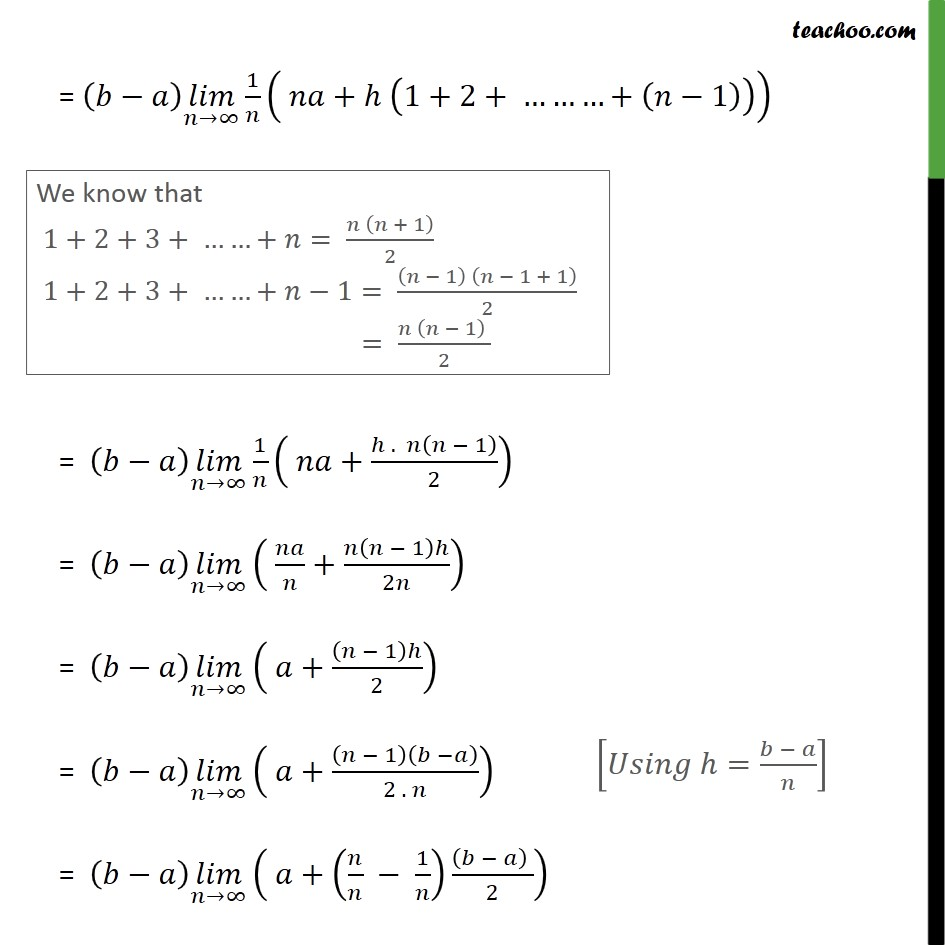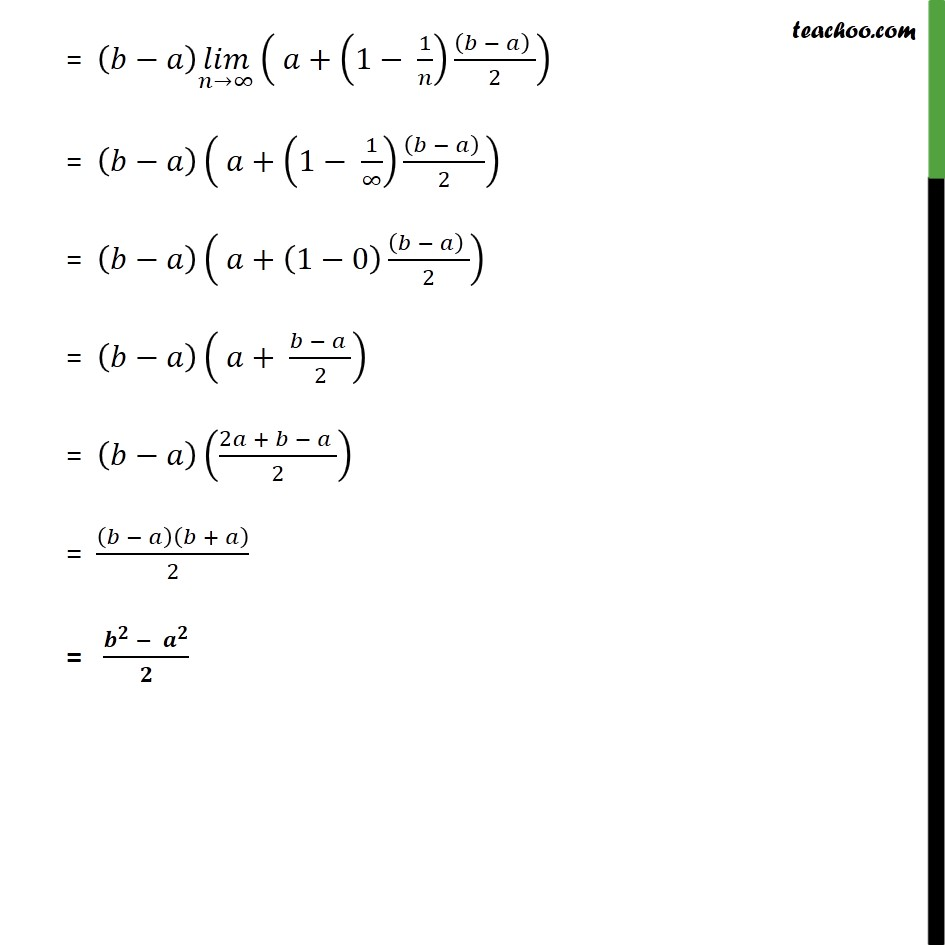1. Chapter 7 Class 12 Integrals
2. Serial order wise
3. Ex 7.8

Transcript

Ex7.8, 1 Putting = = = = = + = + +2 = +2 + 1 = + 1 = uc1 1 + + + +2 + + 1 = uc1 1 + + + +2 + + + 1 = uc1 1 + + + + +2 + + 1 = uc1 1 + 1+2+ + 1 = uc1 1 + . 1 2 = uc1 + 1 2 = uc1 + 1 2 = uc1 + 1 2 . = uc1 + 1 2 = uc1 + 1 1 2 = + 1 1 uc1 2 = + 1 0 2 = + 2 = 2 + 2 = + 2 =

Ex 7.8

Chapter 7 Class 12 Integrals
Serial order wise